Courses

# Test: Design Of Gears (Level - 2)

## 20 Questions MCQ Test Engineering Mechanics | Test: Design Of Gears (Level - 2)

Description
This mock test of Test: Design Of Gears (Level - 2) for Mechanical Engineering helps you for every Mechanical Engineering entrance exam. This contains 20 Multiple Choice Questions for Mechanical Engineering Test: Design Of Gears (Level - 2) (mcq) to study with solutions a complete question bank. The solved questions answers in this Test: Design Of Gears (Level - 2) quiz give you a good mix of easy questions and tough questions. Mechanical Engineering students definitely take this Test: Design Of Gears (Level - 2) exercise for a better result in the exam. You can find other Test: Design Of Gears (Level - 2) extra questions, long questions & short questions for Mechanical Engineering on EduRev as well by searching above.
QUESTION: 1

Solution:
QUESTION: 2

### Consider the following statements:A pinion of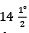pressure angle and 48 involute teeth has a pitch circle diameter of 28.8 cm. It has1. module of 6 mm2. circular pitch of 18 mm3. addendum of 6 mm4. diametra! pitch of 11/113Which of these statements are correct?

Solution: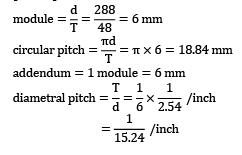addendum = 1 module = 6 mmQUESTION: 3

### A spur gear transmits 10 kW at a pitch line velocity of 10m/s; driving gear has a diameter of 1.0 m. Find the tangential force between the driver and the follower, and the transmitted torque respectively.

Solution:

Power transmitted = Force x Velocity

10 x 103 = Force x 10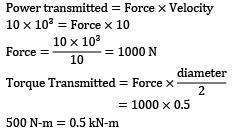= 1000 x 0.5

5000 N-m = 0.5 kN-m

QUESTION: 4

Consider the following statements pertaining to the basic Lewis equation for the strength design of spur gear teeth

1. single pair of teeth participates in power transmission at any instant

2. the tooth is considered as a cantilever beam of uniform strength

4. lewis equation takes into account the inaccuracies of the tooth profile

5. meshing teeth come in contact suddenly

Which of the statements given above are correct?

Solution:
QUESTION: 5

The dynamic load on a gear is due to

1). inaccuracies of tooth spacing

2). irregularities in tooth profile

3). deflection of the teeth under load

4). type of service (i.e. intermittent, one shift per day, continuous per day).

Which of the above statements are correct?

Solution:
*Answer can only contain numeric values
QUESTION: 6

A pinion has 15 teeth and module of 8 mm. The gear ratio is 10 : 1 and the pressure angle is 22.5o. The pinion transmits 500 kW at 1800 rpm then normal load on the tooth will be __________ kN.

Options`

(A) 45

(B) 49

Solution: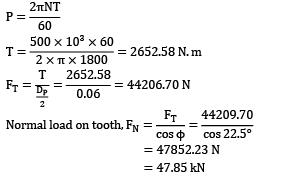= 47852.23 N

= 47.85 kN

*Answer can only contain numeric values
QUESTION: 7

Two mating spur gear have 60 and 180 teeth respectively. The pinion rotates at 1200 rpm and transmits a torque of 40 Nm. The torque transmitted by gear is __________ Nm.

Options`

(A) 120

(B) 120

Solution: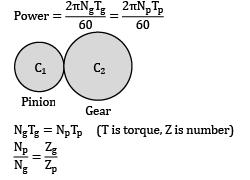NgTg = NpTp (T is torque, Z is number)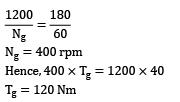Ng = 400 rpm

Hence, 400 x Tg = 1200 x 40

Tg = 120 Nm

*Answer can only contain numeric values
QUESTION: 8

A spur gear has module of 3 mm, number of teeth 16, a face width of 36 mm and a pressure angle of 20o. It is transmitting a power of 3 kW at 20 rev/s. Taking a velocity factor of 1.5 and a form factor of 0.3, the stress in the gear tooth is __________ MPa.

Solution:

Given;

P = 3 kW = 3000 W

N = 20 rps

Y = Form factor = 0.3

b = face width = 36 mm

Cv = velocity factor = 1.5

m = module = 3 mm

Z = teeth = 16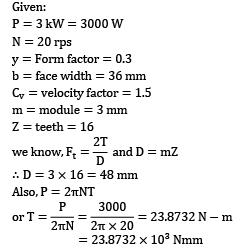∴ D = 3 x 16 = 48 mm

Also, P = 2πNT= 23.8732 x 103Nmm

We know,

(Fd)Lewis ≤ Fb

Ft x Cv ≤ (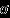bybm)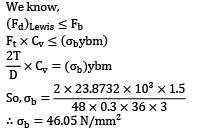b = 46.05 N/mm2

QUESTION: 9

A spur gear has a module of 4 mm, number of teeth 21, a face width 25 mm and a pressure angle of 20o. It is transmitting a power of 4 kW at 25 rev/s. Taking a velocity factor of 1.5 and a form factor of 0.3, the stress in the gear tooth is about

Solution:

Given,P = 4 kW = 4000 W,

N = 25 rps

y = form factor = 0.3

w = 25 mm,

module(m) = 4mm,teeth(Z) = 21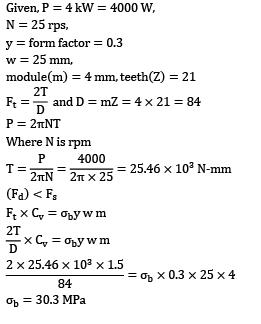P = 2πNT

Where N is rpm(Fd) < />s

Ft x Cv = σby w mσb = 30.3 MPa

QUESTION: 10

A spur gear has a module 3 mm, number of teeth 15 and a pressure angle 20o. It is transmitting torque of 30 Nm at 25 rev/s. The stress on gear tooth is 50 MPa. Taking a velocity factor of 1.4 and a form factor of 0.3. The face width of gear tooth is about

Solution:

Given:T = 30 Nm, m = 3 mm, z = 15,

y = 0.3,Cv = 1.4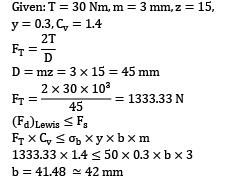D = mz = 3 x 15 = 45 mm(Fd)Lewis ≤ Fs

FT x Cv ≤ σbx y x b x m

1333.33 x 1.4 ≤ 50 x 0.3 x b x 3

b = 41.48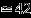mm

QUESTION: 11

The following particulars refer to a spur gear drive, center distance = 200 mm; Ratio of number of teeth on gear to number of teeth on pinion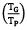= 4 Power transmitted = 50 W; number of teeth on pinion = 18. Pinion speed (Np ) = 1440 rpm. Pressure angle (Φ) = 20o Permissible normal (total) Load = 160 N/mm of face width. The face width in mm is

Solution: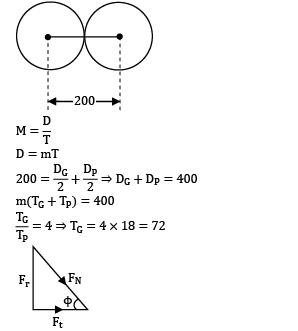m(TG + TP) = 400m(72 + 18) = 400;m = 4.44 = 4.5 mm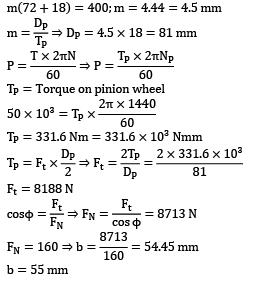Tp = Torque on pinion wheelTp = 331.6 Nm = 331.6 x 103 NmmFt = 8188 Nb = 55 mm

*Answer can only contain numeric values
QUESTION: 12

A spur pinion with module 3 mm and total number of teeth 20 transmits 5 kW of power. The gear rotates at 1000 rpm. The pressure angle of gear tooth is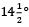.

Assuming that only one pair of teeth is in contact, the total force (in Newton) exerted by a tooth of pinion on the tooth of a mating gear is __________.

Solution: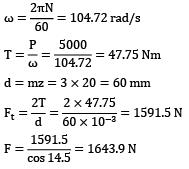d = mz = 3 x 20 = 60 mmQUESTION: 13

A spur pinion rotating at 20 rad/sec and having 20 teeth transmits 20 kW to a gear wheel. The pressure angle is 20o and module is 20 mm. The reaction exerted by the pinion at the center of the shaft is

Solution:

ω = 20 rad/sec, Tp = 20, P = 20 kW

Φ = 20o,m = 20 mm

Normal load exerted by the pinion on the shaft

WN = ?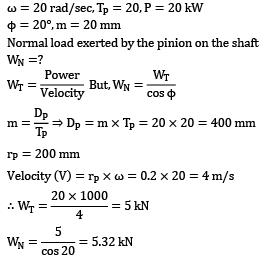rp = 200 mm

Velocity (V) = rp x ω = 0.2 x 20 = 4 m/sQUESTION: 14

A pair of spur pinion and gear made of cast steel (Sut = 110 MPa) and cast iron (Sut = 55 MPa) respectively. The pinion transmits 3274 N at 1250 rpm. The gear ratio is 3 : 1 and the teeth are full involute. Form factor y =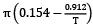.Width is 12 times module. The no. of teeth on pinion is 16, the module is

Solution:

Tp = 16, TG = 48,w = 12 m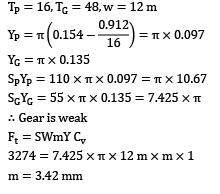YG = π X 0.135

SPYP = 110 X π X 0.097 = π X 10.67

SGYG = 55 x π x 0.135 = 7.425 x π

∴ Gear is weak

Ft = SWmY CV

3274 = 7.425 x π x 12 m x m x 1

m = 3.42 mm

QUESTION: 15

A pinion has 15 teeth and module of 8 mm. The gear ratio is 10 : 1 and pressure angle is 22.5o. The pinion transmits the power 500 kW at 1800 rpm. What will be distance between the centers of gear and pinion and normal load on the tooth?

Solution: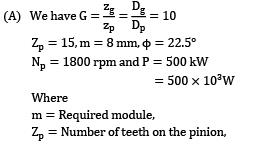Zp = 15,m = 8 mm,Φ = 22.5o

Np = 1800 rpm and P = 500 kW

= 500 x 103W

Where

m = Required module

ZP = Number of teeth on the pinion

Zg = Number of teeth on the gear,

Dp = Pitch circle diameter of the pinion,

Dp = Pitch circle diameter of the gear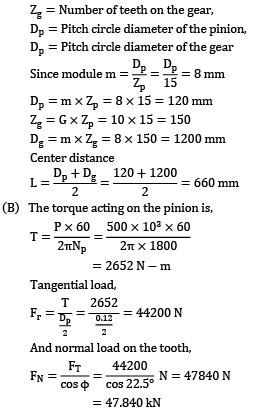Dp = m x Zp = 8 x 15 = 120 mm

Zg = G x Zp = 10 x 15 = 150

Dg = m x Zg = 8 x 150 = 1200 mm

Center distance(B) The torque acting on the pinion is,= 2652 N-mAnd normal load on the tooth= 47.840

QUESTION: 16

A simple gear train is designed with a pinion of 20 teeth and a gear wheel of 60 teeth. They have a pressure angle of 20o and a module of 2 mm. Both the pinion and gear have full depth involute tooth. Due to assembly constraints the center distance was made equal to 80.8 mm what will be the effective pressure angle?

Solution: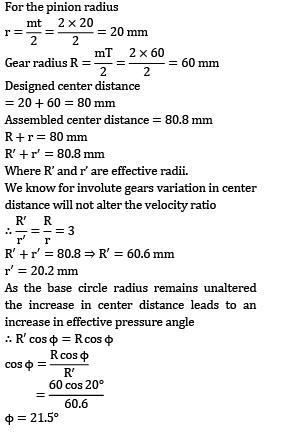Designed center distance

= 20 + 60 = 80 mm

Assembled center distance = 80.8 mm

R + r = 80 mm

R’ + r’ = 80.8 mm

Where R’ and r’ are effective radii.

We know for involute gears variations in center distance will not alter velocity ratioR’ + r’ = 80.8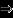R’ = 60.6 mm

r’ = 20.2 mm

As the base circle radius remains unaltered the increase in center distance leads to an increase in effective pressure angle

∴ R’ cos Φ = R cos ΦΦ = 21.5o

*Answer can only contain numeric values
QUESTION: 17

A pair of straight teeth spur gears, having 20o involute full depth teeth is to transmit 12 kW at 300 rpm of the pinion. The speed ratio is 3 : 1. The allowable static stresses for gear of cast iron and pinion of steel are 60 MPa and 105 MPa respectively. Assume the following number of teeth of pinion = 16

Face width = 14 times module

Velocity factor =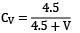Where V = pitch line velocity in m/s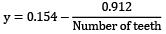.

Tooth form factor y = 丌y

The module of the gear is __________

Solution:

Given

Φ = 20o, Power = 12 kW, Np = 300 rpm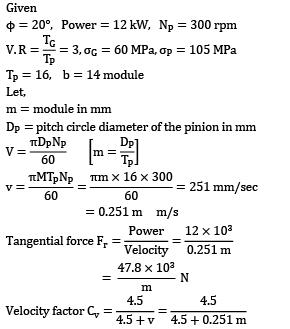Tp = 16, b = 14 module

Let,

m = module in mm

Dp = pitch circle diameter of the pinion in mm= 0.251m m/sQUESTION: 18

Assertion (A): The Lewis equation for design of gear tooth predicts the static load capacity of a cantilever beam of uniform strength.

Reason (R): According to law of gears interchangeability is possible only when gears have same pressure angle and same module.

Solution:

The beam strength of gear teeth is determined from an equation (known as Lewis equation) and the load carrying ability of the toothed gears as determined by this equation gives satisfactory results. In the investigation, Lewis assumed that as the load is being transmitted from one gear to another, it is all given and taken by one tooth, because it is not always safe to assume that the load is distributed among several teeth.

Note:

(i) The Lewis equation is applied only to the weaker of the two wheels (i.e. pinion or gear)

(ii) When both the pinion and the gear are made of the same material, then pinion is the weaker (iii) When the pinion and the gear are made of different materials, then the product of (σW X y) (σo X y) is the deciding factor. The Lewis equation is used to that wheel for which (σW X y) (σo X y) is less.

QUESTION: 19

Pinion 2 in given figure has angular velocity 183.26 rad/s and transmit 2.5 kW to idle gear 3. The teeth cut on 20o the full depth system and their radius are R2 = 25 mm, R3 = 62.5 mm,R4 = 37.5 mm. What is the resultant reaction of gear 3 on its shaft?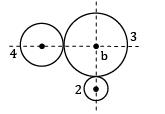(Where T stands for teeth)

Solution:

For pinion 2

P = T2 x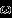2

P = Ft2 x r2 x ω2

Power, P = 2.5 kW

So,P = Ft2 x r2 x ω2

2.5 x 103 = Ft2 x 0.025 x 183.26

Ft2 = 545.67 N

Ft2 = Ft2 x 0.025 x 183.26

Fr2 = Ft2 x tan 20o = 545.67 x tan 20o

Fr2 = 198.607 N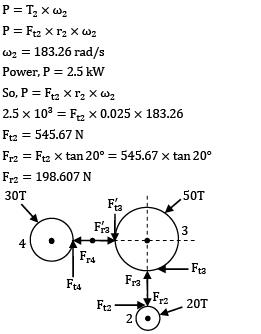For gear 4,

P = Ft4 x r4 x ω4 = Ft4 x r2 x ω2

Ft4 = 545.67 N

So,Ft4 = F’t3 = Ft2 = Ft3 = 545.67 N

Fr4 = F’r3 = Fr2 = Fr3 = 198.607 N

Net force on shaft of gear 3 is: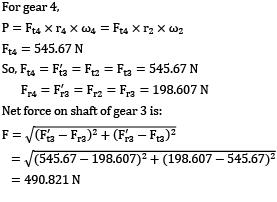= 490.821 N

QUESTION: 20

A spur gear has module of mm, number of teeth 16, a face width of 36 mm and a pressure angle of 20o. It is transmitting power of 3kW at 20 rev/s. Taking velocity factor of 1.5 and a form factor of 0.3, the stress in the gear tooth in MPa is about

Solution:

Beam strength Sb = σb bmY

= σb x 36 x 3 x 0.3

dg = m x tg = 3 x 16 = 48 mm\

Power = 3 kW = 3000 W

3000 = T x ω

3000 = T x 2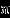n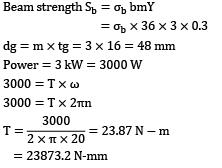= 23873.2 N-mm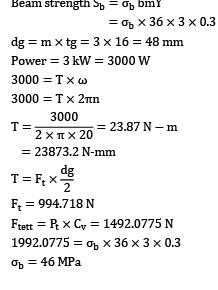Ft = 994.718 N

Ftett = Pt x Cv = 1492.0775 N

1992.0775 = σb x 36 x 3 x 0.3

σb = 46 MPa